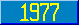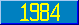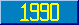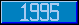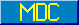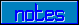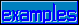## Meaning of numlit

### 7.1.4.4 Meaning of numlit

Note that numlit denotes only nonnegative values. The process of converting the spelling of an occurrence of numlit into its numeric data value consists of the following steps.

1. If the mant has no ".", place one at its right end.
2. If the exp is absent, skip step c.
3. If the exp has a plus or has no sign, move the "." a number of decimal digit positions to the right in the mant equal to the value of the intlit of exp, appending zeros to the right of the mant as necessary. If the exp has a minus sign, move the "." a number of decimal digit positions to the left in the mant equal to the value of the intlit of exp, appending zeros to the left of the mant as necessary.
4. Delete the exp and any leading or trailing zeros of the mant.
5. If the rightmost character is ".", remove it.
6. If the result is empty, make it "0".